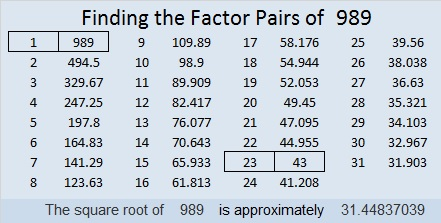# 1449 Christmas Star

If you’ve ever wished you knew the multiplication table better, then make that wish upon this Christmas star. If you use logic and don’t give up,  then you can watch your wish come true!I number the puzzles to distinguish them from one another. That star puzzle is way too big for a factor tree made with its puzzle number:Here’s more about the number 1449:

• Prime factorization: 1449 = 3 × 3 × 7 × 23, which can be written 1449 = 3² × 7 × 23
• 1449 has at least one exponent greater than 1 in its prime factorization so √1449 can be simplified. Taking the factor pair from the factor pair table below with the largest square number factor, we get √1449 = (√9)(√161) = 3√161
• The exponents in the prime factorization are 2, 1, and 1. Adding one to each exponent and multiplying we get (2 + 1)(1 + 1)(1 + 1) = 3 × 2 × 2 = 12. Therefore 1449 has exactly 12 factors.
• The factors of 1449 are outlined with their factor pair partners in the graphic below.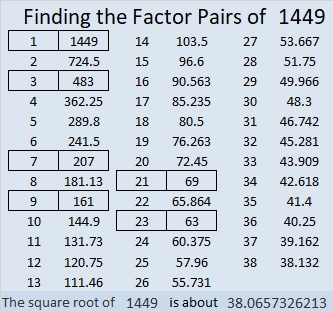1449 is the difference of two squares in 6 different ways:
725² – 724² = 1449
243² – 240² = 1449
107²-100² = 1449
85² – 76² = 1449
45² – 24² = 1449
43² – 20² = 1449

# 1448 Christmas Factor Tree

Here’s a puzzle that looks a little like a Christmas tree. Some of the clues might give you a little bit of trouble. For example, the common factor of 60 and 30 might be 5, 6, or 10. Likewise, the common factor of 8 and 4 might be 1, 2, or 4.

Which factor should you use? Look at all the other clues and use logic. Logic can help you write each of the numbers 1 to 12 in both the first column and the top row so that the given clues and those numbers behave like a multiplication table. Good luck!I have to number every puzzle. It won’t help you solve the puzzle, but here are some facts about the number 1448:

The number made by its last two digits, 48, is divisible by 4, so 1448 is also divisible by 4. That fact can give us the first couple of branches of 1448’s factor tree: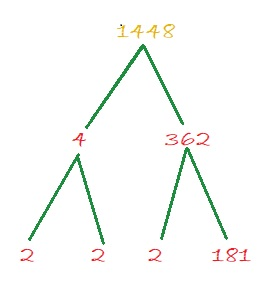• 1448 is a composite number.
• Prime factorization: 1448 = 2 × 2 × 2 × 181, which can be written 1448 = 2³ × 181
• 1448 has at least one exponent greater than 1 in its prime factorization so √1448 can be simplified. Taking the factor pair from the factor pair table below with the largest square number factor, we get √1448 = (√4)(√362) = 2√362
• The exponents in the prime factorization are 3 and 1. Adding one to each exponent and multiplying we get (3 + 1)(1 + 1) = 4 × 2 = 8. Therefore 1448 has exactly 8 factors.
• The factors of 1448 are outlined with their factor pair partners in the graphic below.1448 is also the hypotenuse of a Pythagorean triple:
152-1440-1448 which is 8 times (19-180-181)

# 1447 Christmas Light Puzzle

If you’ve ever had a string of lights go out because ONE bulb went bad, it can be a very frustrating puzzle to figure out which light is causing the problem.

This is not that kind of puzzle. For this one, you just need to figure out where to put the numbers from 1 to 12 in both the first column and the top row so that the given clues are the products of those numbers. There is only one solution, and if you always use logic, it will not be a frustrating puzzle to solve.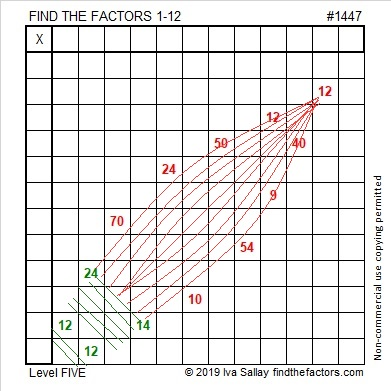I gave that puzzle the puzzle number 1447. That number won’t help you solve the puzzle, but here are some facts about it anyway:

• 1447 is a prime number.
• Prime factorization: 1447 is prime.
• 1447 has no exponents greater than 1 in its prime factorization, so √1447 cannot be simplified.
• The exponent in the prime factorization is 1. Adding one to that exponent we get (1 + 1) = 2. Therefore 1447 has exactly 2 factors.
• The factors of 1447 are outlined with their factor pair partners in the graphic below.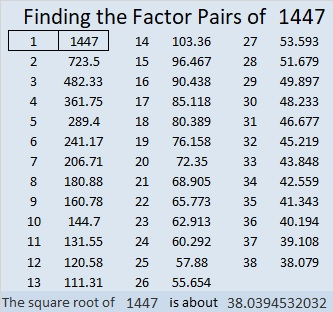How do we know that 1447 is a prime number? If 1447 were not a prime number, then it would be divisible by at least one prime number less than or equal to √1447. Since 1447 cannot be divided evenly by 2, 3, 5, 7, 11, 13, 17, 19, 23, 29, 31, or 37, we know that 1447 is a prime number.

1447 is also the difference of two consecutive squares:
724² – 723² = 1447

# 1446 Peppermint Stick

Red and green striped peppermint sticks are often seen in stores and homes in December. Can you lick this peppermint stick puzzle or will you let it lick you?The puzzle number was 1446. Here are a few facts about that number:

• 1446 is a composite number.
• Prime factorization: 1446 = 2 × 3 × 241
• 1446 has no exponents greater than 1 in its prime factorization, so √1446 cannot be simplified.
• The exponents in the prime factorization are 1, 1, and 1. Adding one to each exponent and multiplying we get (1 + 1)(1 + 1)(1 + 1) = 2 × 2 × 2 = 8. Therefore 1446 has exactly 8 factors.
• The factors of 1446 are outlined with their factor pair partners in the graphic below.1446 is also the hypotenuse of a Pythagorean triple:
720-1254-1446 which is 6 times (120-209-241)

# 1444 Christmas Wrapping Paper

A fourth of the products in this multiplication table puzzle are already there just because I wanted the puzzle to have a wrapping paper pattern. Can you figure out what the factors are supposed to be and what all the other products are?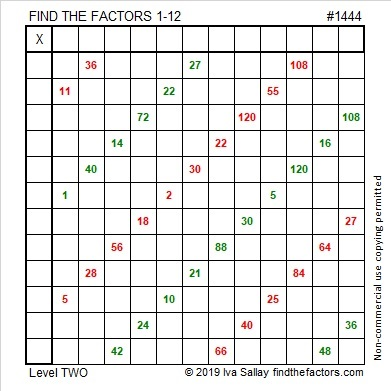• 1444 is a composite number and a perfect square.
• Prime factorization: 1444 = 2 × 2 × 19 × 19, which can be written 1444 = 2²× 19²
• 1444 has at least one exponent greater than 1 in its prime factorization so √1444 can be simplified. Taking the factor pair from the factor pair table below with the largest square number factor, we get √1444 = (√4)(√361) = (2)(19) = 38
• The exponents in the prime factorization are 2 and 2. Adding one to each exponent and multiplying we get (2 + 1)(2 + 1) = 3 × 3 = 9. Therefore 1444 has exactly 9 factors.
• The factors of 1444 are outlined with their factor pair partners in the graphic below.Square number 1444 looks a lot like another square number, 144.
If we keep adding 4’s to the end, will we continue to get square numbers?
No.

However, in different bases, 1444 looks like several other square numbers:
It’s 484 in BASE 18,
400 in BASE 19,
169 in BASE 35,
144 in BASE 36,
121 in BASE 37, and
100 in BASE 38.

# 1332 Yet Another Christmas Tree

Here is yet another Christmas tree for you to enjoy this holiday season.

Print the puzzles or type the solution in this excel file:10-factors-1321-1332

Here are a few facts about the number 1332:

• 1332 is a composite number.
• Prime factorization: 1332 = 2 × 2 × 3 × 3 × 37, which can be written 1332 = 2² × 3² × 37
• The exponents in the prime factorization are 2, 2 and 1. Adding one to each and multiplying we get (2 + 1)(2 + 1)(1 + 1) = 3 × 3 × 2 = 18. Therefore 1332 has exactly 18 factors.
• Factors of 1332: 1, 2, 3, 4, 6, 9, 12, 18, 36, 37, 74, 111, 148, 222, 333, 444, 666, 1332
• Factor pairs: 1332 = 1 × 1332, 2 × 666, 3 × 444, 4 × 333, 6 × 222, 9 × 148, 12 × 111, 18 × 74 or 36 × 37
• Taking the factor pair with the largest square number factor, we get √1332 = (√36)(√37) = 6√37 ≈ 36.49658

Here are a couple of factor trees for 1332:Since 36 × 37 = 1332, we know that 1332 is the sum of the first 36 even numbers. (The first 36 numbers add up to the infamous 666, and 2 times 666 is 1332.)

Because 1332 is divisible by both 3 and 37, it has several repdigits as factors, 111, 222, 333, 444, and 666.

1332 is the sum of four consecutive prime numbers:
317 + 331 + 337 + 347 = 1332

1332 is the sum of two square numbers:
36² + 6² = 1332

1332 is the hypotenuse of a Pythagorean triple:
432-1260-1332 which is 36 times (12-35-37)
It can also be calculated from 2(36)(6), 36² – 6², 36² + 6²

# 1328 Christmas Tree

Can you figure out where to put the numbers from 1 to 10 in both the first column and the top row so that the lights on this Christmas tree work properly?

Print the puzzles or type the solution in this excel file:10-factors-1321-1332

Now I’ll share some facts about the puzzle number, 1328:

• 1328 is a composite number.
• Prime factorization: 1328 = 2 × 2 × 2 × 2 × 83, which can be written 1328 = 2⁴ × 83
• The exponents in the prime factorization are 4 and 1. Adding one to each and multiplying we get (4 + 1)(1 + 1) = 5 × 2 = 10. Therefore 1328 has exactly 10 factors.
• Factors of 1328: 1, 2, 4, 8, 16, 83, 166, 332, 664, 1328
• Factor pairs: 1328 = 1 × 1328, 2 × 664, 4 × 332, 8 × 166, or 16 × 83
• Taking the factor pair with the largest square number factor, we get √1328 = (√16)(√83) = 4√83 ≈ 36.44173

Because 28 is divisible by 4, but not by 8, and 3 (the digit before the 28) is an odd number, I know that 1328 is divisible by 8. I can use that fact to make this simple factor tree:1328 is the difference of two squares three different ways:
333² – 331² = 1328
168² – 164² = 1328
87² – 79²  = 1328

# 993 Christmas Angel

There are 21 clues in this Christmas Angel puzzle. Will it be easy or difficult for you to solve? That is part of the mystery. As always, there is only one solution. Can you find it?Print the puzzles or type the solution in this excel file: 12 factors 993-1001

When is 993 a palindrome?
It is 5B5 in BASE 13 (B is 11 base 10) because 5(169) + 11(13) + 5(1) = 993,
313 in BASE 18 because 3(18²) + 1(18) + 3(1) = 993,
and it is repdigit 111 in BASE 31 because 31² + 31¹ + 31⁰ = 993

• 993 is a composite number.
• Prime factorization: 993 = 3 × 331
• The exponents in the prime factorization are 1 and 1. Adding one to each and multiplying we get (1 + 1)(1 + 1) = 2 × 2 = 4. Therefore 993 has exactly 4 factors.
• Factors of 993: 1, 3, 331, 993
• Factor pairs: 993 = 1 × 993 or 3 × 331
• 993 has no square factors that allow its square root to be simplified. √993 ≈ 31.5119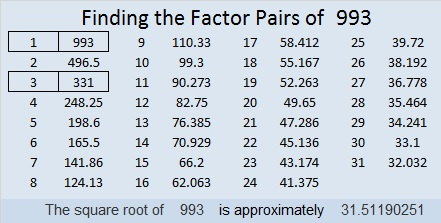# 992 Christmas Factor Tree

Artificial Christmas trees have to be assembled. Sometimes the assembly is easy, and sometimes it is frustrating.

This Christmas tree puzzle can be solved using LOGIC and an ordinary multiplication table, but there’s a good chance it will frustrate you. Go ahead and try to solve it!Print the puzzles or type the solution in this excel file: 10-factors-986-992

The number 992 also can make a nice looking, well-balanced factor tree:992 is the product of two consecutive numbers: 31 × 32 = 992.
Because of that fact, 992 is the sum of the first 31 EVEN numbers:
2 + 4 + 6 + 8 + 10 + . . . + 54 + 56 + 58 + 60 + 62 = 992

992 is palindrome 212 in BASE 22 because 2(22²) + 1(22) + 2(1) = 922. That was a lot of 2’s and 1’s in that fun fact!

• 992 is a composite number.
• Prime factorization: 992 = 2 × 2 × 2 × 2 × 2 × 31, which can be written 992 = 2⁵ × 31
• The exponents in the prime factorization are 5 and 1. Adding one to each and multiplying we get (5 + 1)(1 + 1) = 6 × 2 = 12. Therefore 992 has exactly 12 factors.
• Factors of 992: 1, 2, 4, 8, 16, 31, 32, 62, 124, 248, 496, 992
• Factor pairs: 992 = 1 × 992, 2 × 496, 4 × 248, 8 × 124, 16 × 62, or 31 × 32
• Taking the factor pair with the largest square number factor, we get √992 = (√16)(√62) = 4√62 ≈ 31.49603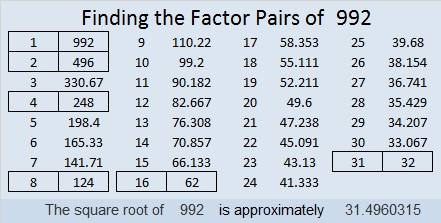# 989 Christmas Bells at Eventide

I made this puzzle with silver clues to look like a bell. I thought dark blue looked best as a background color with the silver numbers. The puzzle reminds me of a bell in the evening. For the fun of it, I googled Christmas Bells in the evening to see if any poems or songs came up.Print the puzzles or type the solution in this excel file: 10-factors-986-992

I was very surprised that Google found a very old song called Christmas Bells at Eventide. I did not know that such a song existed. Eventide means the same thing as evening. You can listen to the song below.

This is my 989th post.

The number 989 is a palindrome in base 10. What about any other bases?
It’s 373 in BASE 17 because 3(17²) + 7(17) + 3(1) = 989,
252 in BASE 21 because 2(21²) + 5(21) + 2(1) = 989, and
1C1 in BASE 26 (C is 12 base 10) because 1(26²) + 12(26) + 1(1) = 989

• 989 is a composite number.
• Prime factorization: 989 = 23 × 43
• The exponents in the prime factorization are 1 and 1. Adding one to each and multiplying we get (1 + 1)(1 + 1) = 2 × 2 = 4. Therefore 989 has exactly 4 factors.
• Factors of 989: 1, 23, 43, 989
• Factor pairs: 989 = 1 × 989 or 23 × 43
• 989 has no square factors that allow its square root to be simplified. √989 ≈ 31.44837